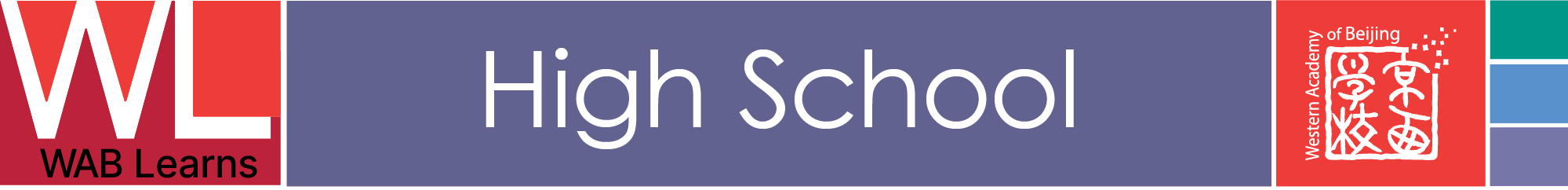# Theory of Knowledge: Mathematics

resources for TOK at WAB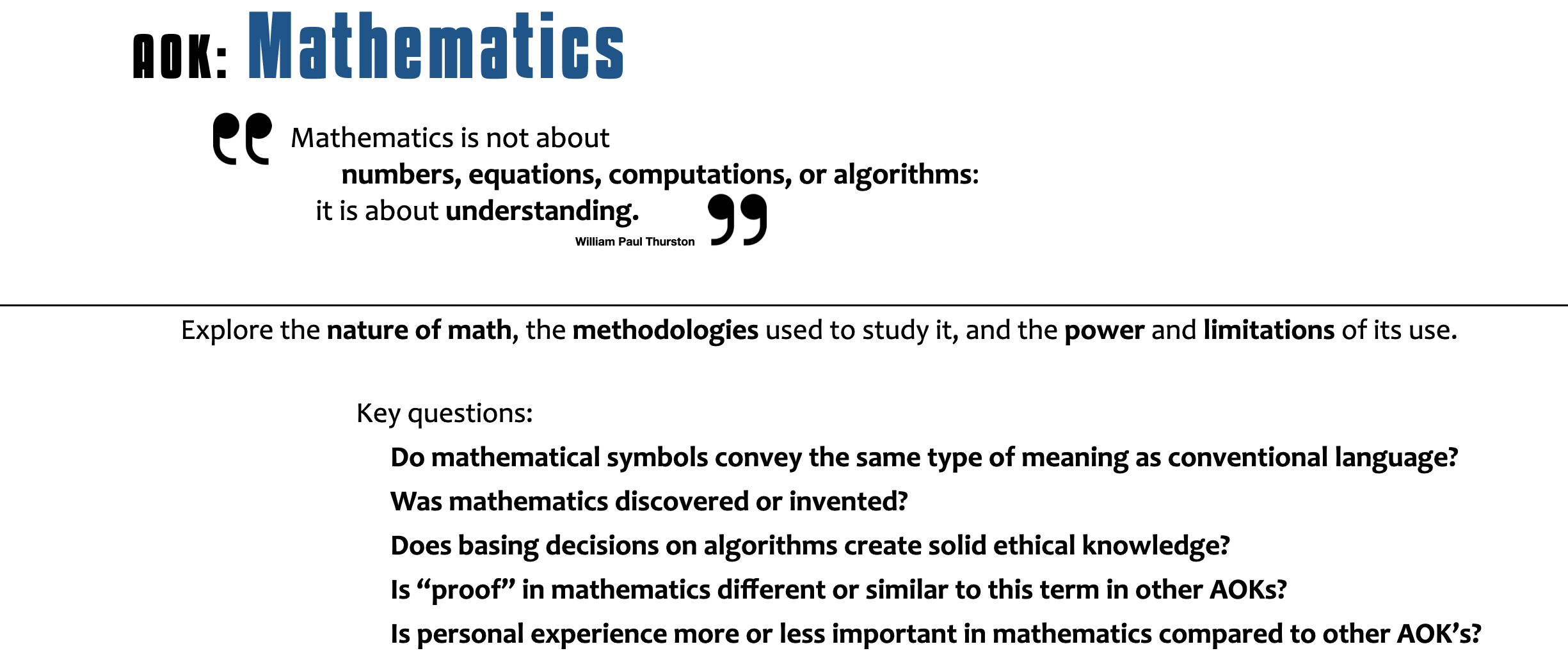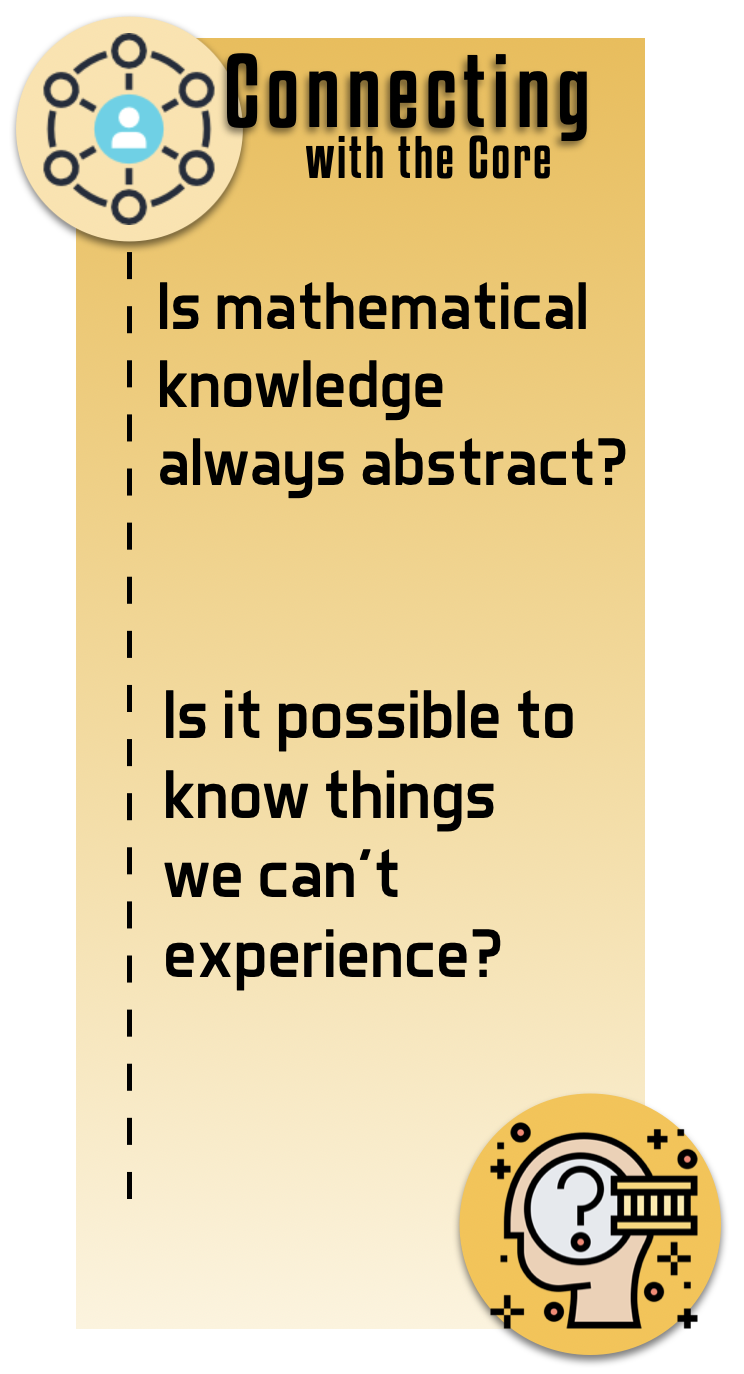## Concepts in Math

Does mathematics only yield knowledge about the real world when it is combined with other areas of knowledge?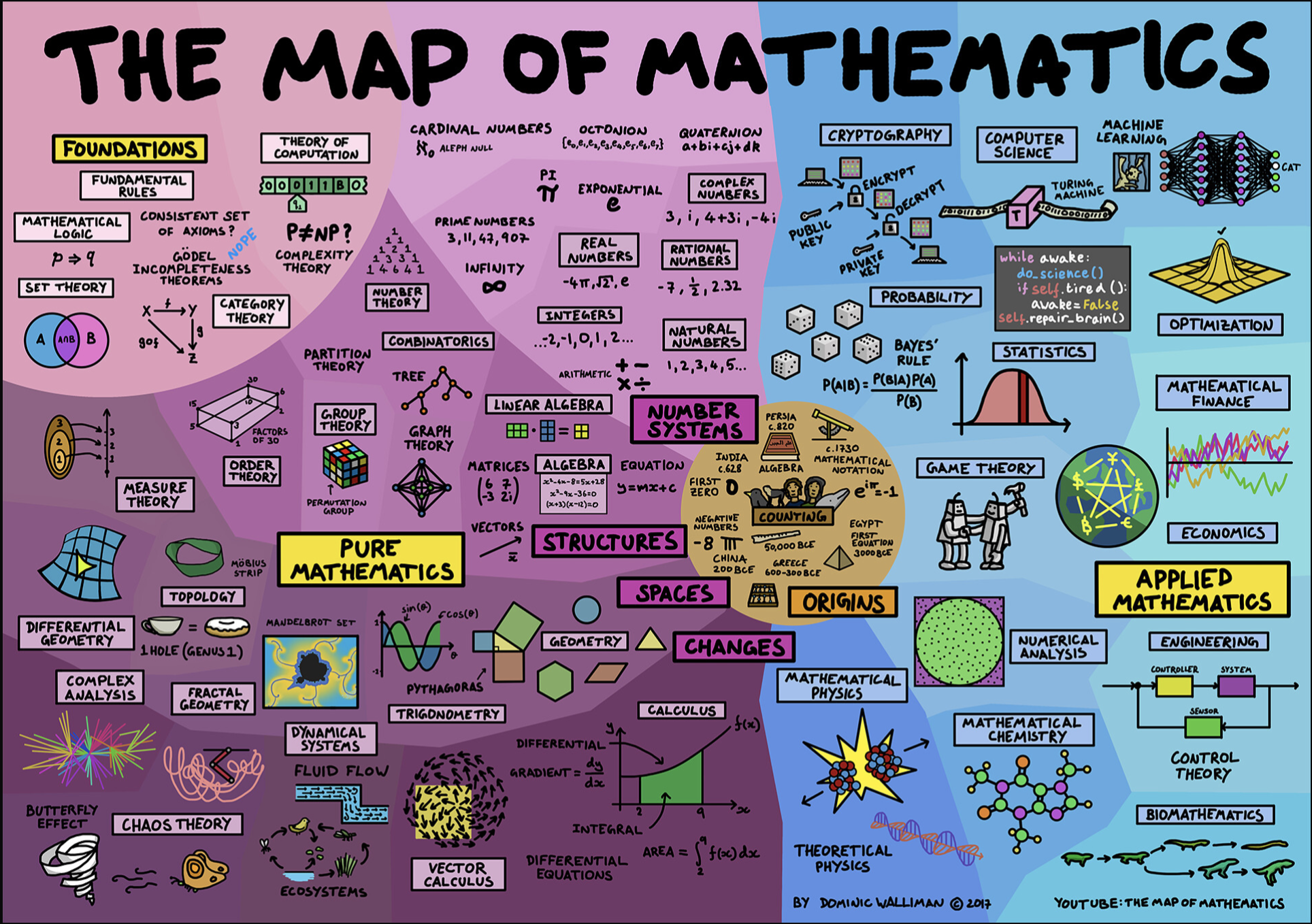SOURCE

How significant have notable individuals been in shaping the nature and development of mathematics as an area of knowledge?

Is mathematical knowledge always abstract?

​Is mathematical reasoning different from scientific reasoning or reasoning in other areas of knowledge?

What is "proof" to mathematicians?  Is this different from proof in other AOKs?

Is mathematics invented or discovered?   Is mathematics better defined by its subject matter or its method?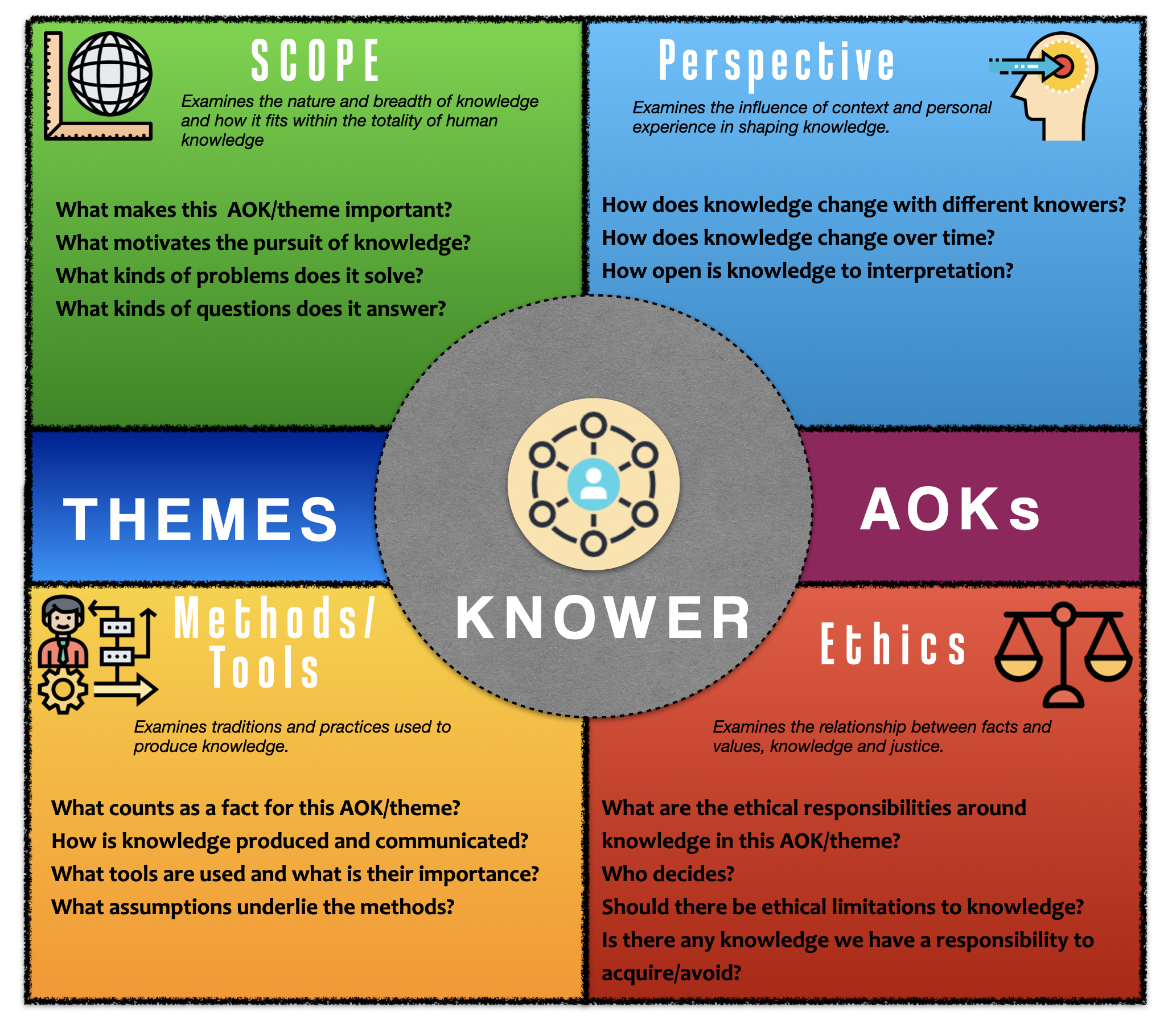## Things that make you go hmmm....

Does emotion play a role in mathematical knowledge?

If numbers and mathematical objects are mere mental constructs, something invented by us, then why does mathematics work so well and seem to describe the world? On the other hand, if mathematical entities are "out there" in some sense waiting to be discovered, then what is their status and how do we get knowledge of them?

Is math objective?    (You can find O'Neill's Weapons of Math Destruction and Noble's Algorithms of Oppression in the library)

Are there limits to mathematical knowledge?

Can math add to other areas of knowledge?

Is it ethically justifiable for academic researchers to spend time doing research that does not have useful applications?